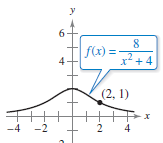×
Get Full Access to Calculus: Early Transcendental Functions - 6 Edition - Chapter 3.3 - Problem 75
Get Full Access to Calculus: Early Transcendental Functions - 6 Edition - Chapter 3.3 - Problem 75

×

# Famous Curves In Exercises 7578, find an equation of the tangent line to the graph atISBN: 9781285774770 141

## Solution for problem 75 Chapter 3.3

Calculus: Early Transcendental Functions | 6th Edition

• Textbook Solutions
• 2901 Step-by-step solutions solved by professors and subject experts
• Get 24/7 help from StudySoup virtual teaching assistantsCalculus: Early Transcendental Functions | 6th Edition

4 5 1 240 Reviews
30
5
Problem 75

Famous Curves In Exercises 75–78, find an equation of the tangent line to the graph at the given point. (The graphs in Exercises 75 and 76 are called Witches of Agnesi. The graphs in Exercises 77 and 78 are called serpentines.)Text Transcription:

Step-by-Step Solution:

Step 1 of 5) Divergence in Three Dimensions The divergence of a vector field F = M(x, y, z)i + N(x, y, z)j + P(x, y, z)k is the scalar function

Step 2 of 2

##### ISBN: 9781285774770

This full solution covers the following key subjects: . This expansive textbook survival guide covers 134 chapters, and 10738 solutions. The answer to “?Famous Curves In Exercises 75–78, find an equation of the tangent line to the graph at the given point. (The graphs in Exercises 75 and 76 are called Witches of Agnesi. The graphs in Exercises 77 and 78 are called serpentines.) Text Transcription:” is broken down into a number of easy to follow steps, and 43 words. The full step-by-step solution to problem: 75 from chapter: 3.3 was answered by , our top Calculus solution expert on 11/14/17, 10:53PM. Calculus: Early Transcendental Functions was written by and is associated to the ISBN: 9781285774770. This textbook survival guide was created for the textbook: Calculus: Early Transcendental Functions, edition: 6. Since the solution to 75 from 3.3 chapter was answered, more than 216 students have viewed the full step-by-step answer.

Unlock Textbook Solution